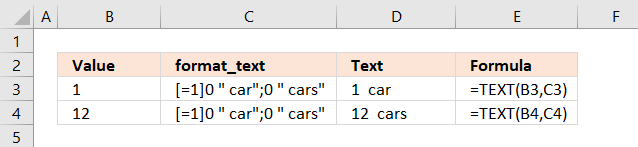Author: Oscar Cronquist Article last updated on October 01, 2021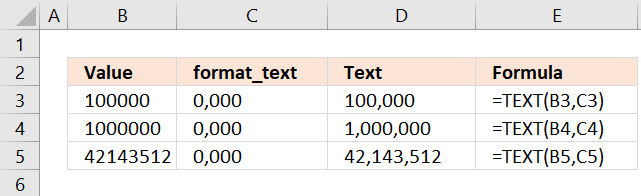This article demonstrates how to use the TEXT function in great detail. The formula in cell D3 formats the number in cell B3 based on the formatting text in cell C3 and returns the result to cell D3.

Formula in cell D3:

=TEXT(B3,C3)

The formatting text lets you format numbers as you prefer, 0,000 formats numbers by inserting a comma after every third digit. You have a plethora of formatting characters you can use all described below.

### Excel function syntax

TEXT(value, format_text)

Converts a value to text in a specific number format.

### Arguments

 value The string you want to format. You can use a cell reference here or use a text string. format_text Formatting code allowing you to change the way, for example, a date or a number is displayed to the Excel user.

## 1. How to create your own custom formatting - TEXT function

The TEXT function lets you easily create your own custom formats, there are four parts shown in the picture below.

You can use the semicolon to separate different settings for different values, the zero is a character symbol (among others) for digits and the @ is a character symbol for text values.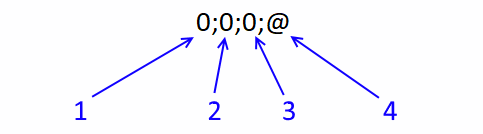1. Positive values
2. Negative values
3. Zero values
4. Text values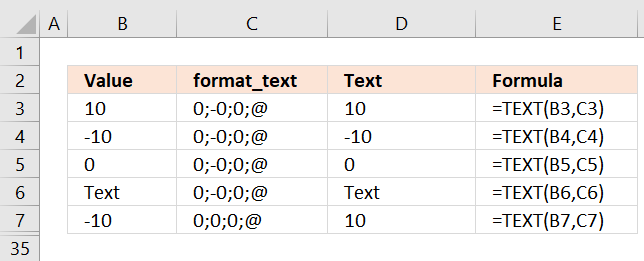The format_ text argument is 0;-0;0;@ Positive numbers are returned positive, no change.

Formula in cell D3:

=TEXT(B3, C3)

The format_text argument is specified in cell C3 in this example, however, you can type it in the second argument as well. Like this:

=TEXT(B3,"0;-0;0;@")

You can ignore the semicolons if you want to format all values equally.

### 1.1 How to convert negative numbers to positive numbers?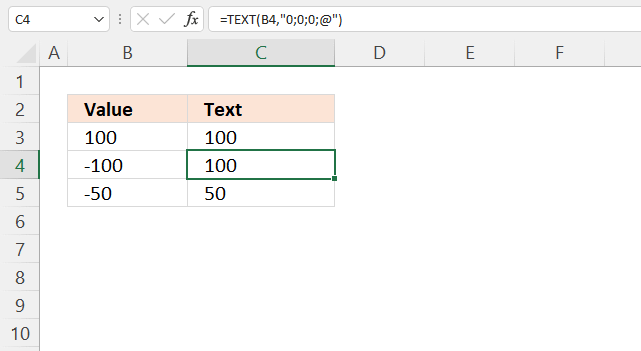The image above shows you a negative number in cell B4, the formula in cell C4 removes the sign.

The second container lets you format negative numbers, this means that you can use the TEXT function to remove the minus sign from negative numbers.

=TEXT(B3,"0;0;0;@")

The bolded zero in the formula above shows the container for negative numbers. The minus sign is removed, negative numbers are converted to positive numbers.

### 1.2 How to convert positive numbers to negative numbers?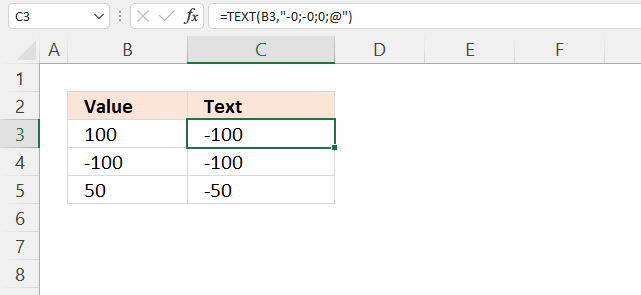The picture above demonstrates a formula in cell C4 that converts positive numbers to negative numbers.

=TEXT(B3,"-0;-0;0;@")

The first container in the formula above (bolded) lets you format positive numbers, the minus sign formats positive numbers as negative numbers.

### 1.3 How to change positive numbers to negative numbers and vice versa?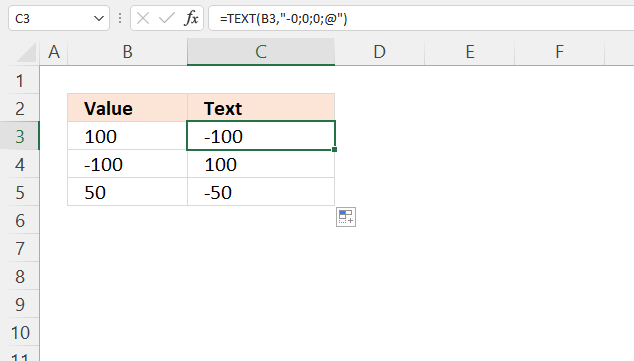The image above shows the TEXT function in cell C4, it changes positive numbers to negative and negative numbers to positive.

=TEXT(B3,"-0;0;0;@")

The first and second container (bolded) formats numbers as explained above. You can also multiply the number with -1, like this:

=B3*-1

This is a lot easier than using the TEXT function. The asterisk multiples the number in cell B3 with -1 which changes the sign, in other words, positive numbers become negative and vice versa.

## 2. Formatting codes you can use

Note that you cant perform arithmetic operations to a value the TEXDT function has calculated. Excel handles these values as text values even if all value characters are digits.

### 2.1 How to insert 0 zeros to the right of the decimal point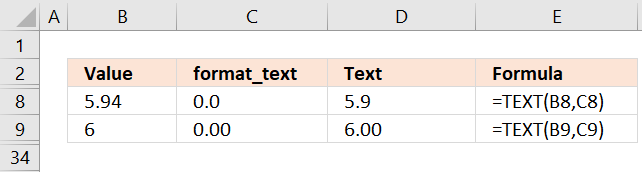The decimal point lets you round numbers, it also inserts additional decimals even if it is not needed.

Formula in cell D9:

=TEXT(B9,C9)

The format_text argument is 0.00. This adds additional zeros to the right of the decimal point, even if they are not needed.

For example, 6 becomes 6.00. I will show you below how to round numbers, follow the link to get to the section below.

### 2.2 How to separate digits by a commaThe comma lets you separate numbers into any number of digits. The example above shows how to separate every three digits with a comma.  This makes large numbers easier to read.

Formula in cell D3:

=TEXT(B3, C3)

The format_text argument is "0,000", this separates text with a comma every third digit.

### 2.3 How to add 0 (zeros) to the right and left of the decimal point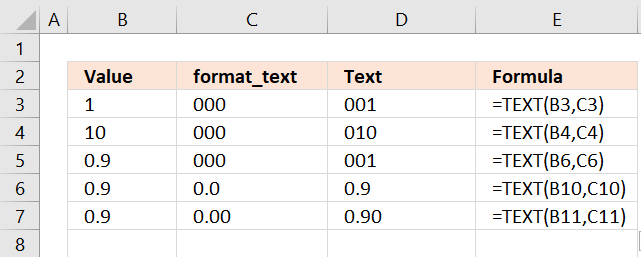The image above shows different formatting patterns using the 0 (zero) character. It allows you to add 0 (zeros) before and after the decimal point.

Formula in cell D3:

=TEXT(B3, C3)

The format_text argument is "000", this returns a number with leading zeros if the number is smaller than 100.

How to type multiple zeros in one cell?
This is not working for me, Excel shortens the digits to only a single 0 (zero).Type the zeros like this: '000
Then press Enter.

### 2.4 Hashtag character lets you hide extra zeros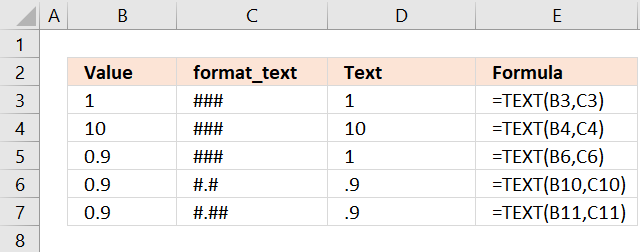The image above shows how to use hashtags in the TEXt function. The hashtag is a digit symbol and 0's (zeros) to the left of the decimal point is not shown.

Formula in cell D3:

=TEXT(B3, C3)

### 2.5 How to align numbers by the decimal point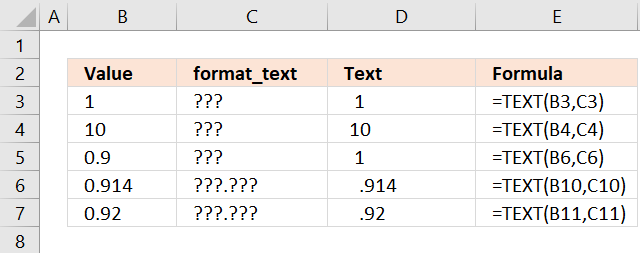The image above shows what happens if you use the ? (question mark) as a placeholder in the TEXT function. It aligns numbers based on the decimal point and hides 0's (zeros) if possible.

Formula in cell D3:

=TEXT(B3, "???")

You can also use the ? (question mark) to create fractions based on a decimal number.

### 2.6 How to create a percentage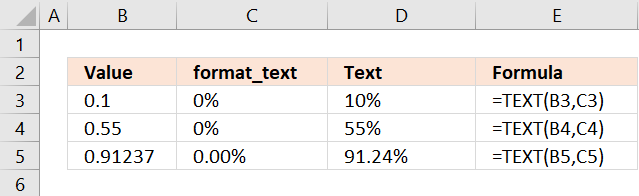The picture above demonstrates how to format numbers as percentages.

Formula in cell D3:

=TEXT(B3, "0%")

The decimal point and the 0 (zero) lets you format the percentage number.

### 2.7 How to format a number using the Scientific notation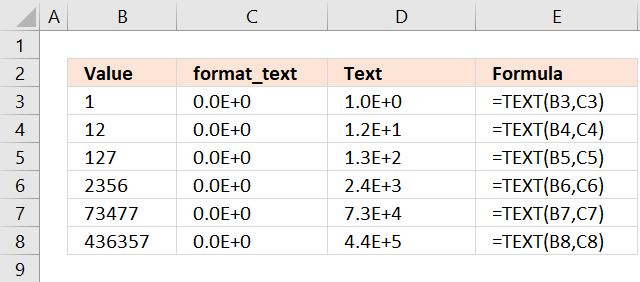The image shows how to format numbers using the scientific format. It allows you to show very large numbers (many digits) or very small numbers in a decimal form.

Formula in cell D3:

=TEXT(B3, "0.0E+0")

E+ E- e+ e- - Scientific format.

E or e represents "value times ten raised to the power of". Superscripted exponents can be shown in Excel but not as a result of a formula. As far as I know, you need to format the output to create a superscripted exponent.

 Number Scientific format x^n 2 2E+0 2*10^0 200 2E+2 2*10^2 0.2 2E-1 2*10^-1 0.02 2E-2 2*10^-2 0.002 2E-3 2*10^-3

### 2.8 How to use text in the TEXT function - @ At character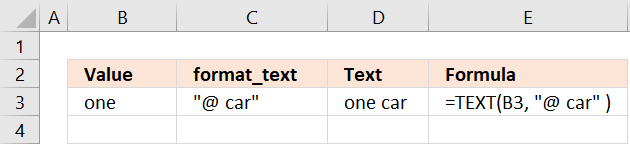The image above shows how to use the "at" character with text values in the TEXT function.

Formula in cell D3:

=TEXT(B3, "@ car")

The at character is a placeholder for text values, in this case, the text value in cell B3. @ = "one", "@ car" becomes "one car" in cell D3.

@ - Text characters

### 2.9 How to work with text and numbers in the TEXT function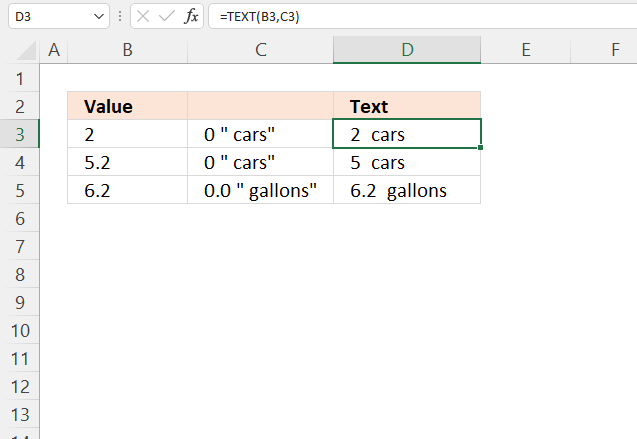Formula in cell D3:

=TEXT(B3, 0 " cars")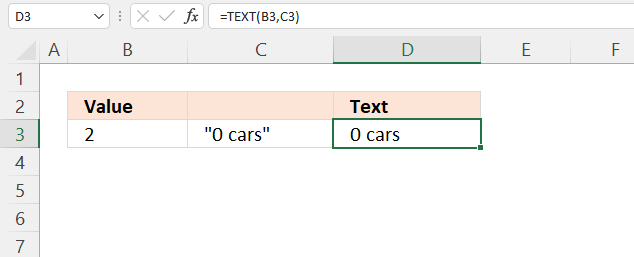"Text" - Characters enclosed by quotation marks show the characters and digits.

### 2.10 Date formats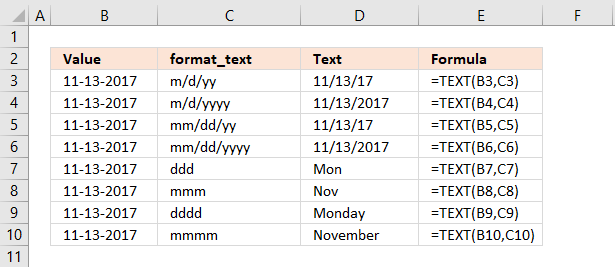The image above demonstrates how to work with dates using the TEXT function.

 Placeholder Source date Result Description m 1-13-2017 1 Extracts month as a number without a leading zero. mm 1-13-2017 01 Extracts month as a number with a leading zero. mmm 1-13-2017 Jan Extracts month as a three-letter abbreviation. mmmm 1-13-2017 January Extracts full month name. d 11-3-2017 3 Extracts day as a number without a leading zero. dd 11-3-2017 03 Extracts day as a number with a leading zero. ddd 11-13-2017 Mon Extracts weekday name as a three-letter abbreviation. dddd 11-13-2017 Monday Extracts full weekday name. yy 11-3-2017 17 Extracts year as a two-digit number. yyyy 11-3-2017 2017 Extracts full year.

#### 2.10.1 How to extract the month number without a leading zero?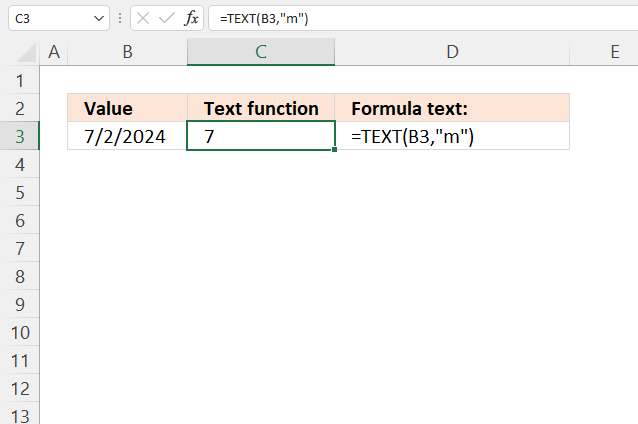The image above shows how to extract the month number without a leading zero using the TEXT function, the source date is in cell B3.

1 - January
2 - February
3 - March
4 - April
5 - May
6 - June
7 - July
8 - August
9 - September
10 - October
11 - November
12- December

Formula in cell C3:

=TEXT(B3, "m")

m - Month number without a leading zero

#### 2.10.2 How to extract the month number with a leading zero?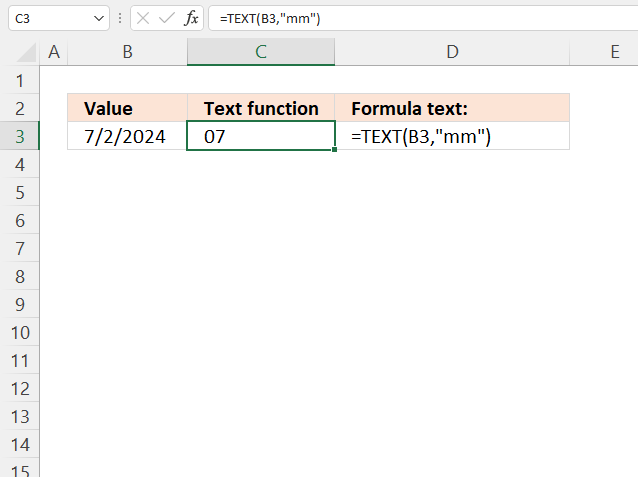The image above shows how to extract the month number with a leading zero using the TEXT function, the source date is in cell B3.

01 - January
02 - February
03 - March
04 - April
05 - May
06 - June
07 - July
08 - August
09 - September
10 - October
11 - November
12- December

Formula in cell C3:

=TEXT(B3, "mm")

mm - Month number with a leading zero

#### 2.10.3 How to extract the month as a three-letter abbreviation?mmm - Month as an abbreviation, Jan, Feb, Mar, Apr, May, Jun, Jul, Aug, Sep, Oct, Nov and Dec.

#### 2.10.4 How to extract the full month from a date?mmmm - Full month name.

#### 2.10.5 How to extract the day as a number without a leading zero from a date?d- Day as a number without a leading zero

#### 2.10.6 How to extract the day as a number with a leading zero from a date?dd - Day as a number with a leading zero when necessary

#### 2.10.6 How to extract the weekday as a three-letter abbreviation from a date?ddd - Day as an abbreviation, Sun, Mon, Tue, Wed, Thu, Fri and Sat.

#### 2.10.6 How to extract the full weekday name from a date?dddd - Full day name.

#### 2.10.6 How to extract the year as a two-digit number from a date?yy - Year as a two-digit number.

#### 2.10.6 How to extract the full year from a date?yyyy - Year as a four-digit number.

### 2.11 Time formats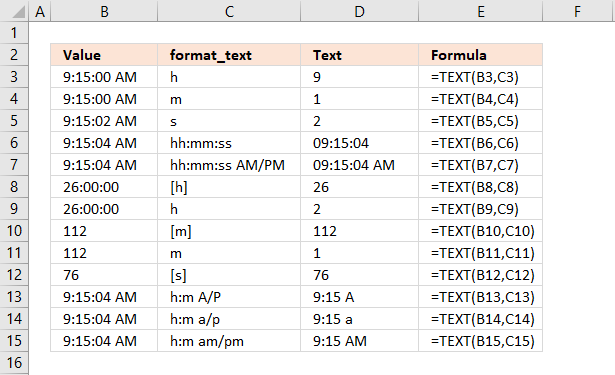h - Hour as a digit without a leading zero.

[h] - Shows more than 24 hours.

hh - Hour as a digit with a leading zero when needed.

m - Minute without a leading zero.

[m] - Shows more than 60 minutes.

mm - Minute with a leading zero when necessary.

s - Second without a leading zero.

[s] - Shows more than 60 seconds.

ss - Seconds with a leading zero when necessary.

AM/PM - Shows the time of 12-hour hours.

am/pm - See above.

A/P - See above.

a/p - See above.

The image above demonstrates how to work with time values using the TEXT function.

 Placeholder Source date Result Description h 9:15:04 AM 9 Hour as a digit without a leading zero. [h] 1-13-2017 01 Extracts month as a number with a leading zero. hh 1-13-2017 Jan Extracts month as a three-letter abbreviation. m 1-13-2017 January Extracts full month name. [m] 11-3-2017 3 Extracts day as a number without a leading zero. mm 11-3-2017 03 Extracts day as a number with a leading zero. s 11-13-2017 Mon Extracts weekday name as a three-letter abbreviation. [s] 11-13-2017 Monday Extracts full weekday name. ss 11-3-2017 17 Extracts year as a two-digit number. AM/PM 11-3-2017 2017 Shows the time of 12-hour hours. am/pm 11-3-2017 2017 See above. A/P 11-3-2017 2017 See above. a/p 11-3-2017 2017 See above.

### 2.12 Why isn't the TEXT function working?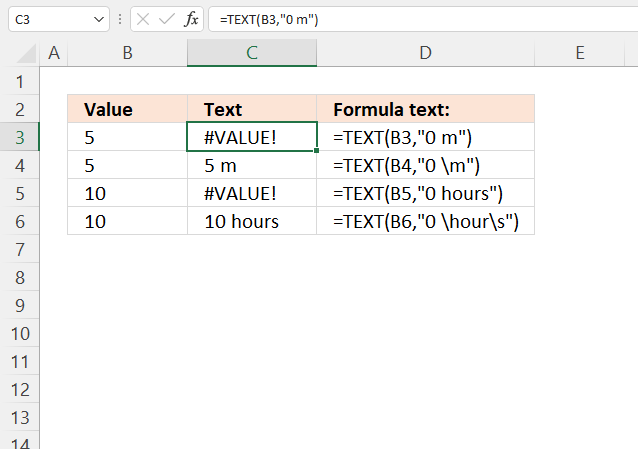The TEXT function returns a #VALUE! error if you have a placeholder character in the second argument, see example formula in cells C3 and C4 above. Use the backslash to escape placeholder characters.

Formula in cell D3:

=TEXT(B3, "0 m")

Character m is a placeholder character for a month number, however, we want to use it as the abbreviation for the meter (metric system) in the example above in cell C3. The formula returns a #VALUE! error.

Cell C4 has a backslash character before the m character.

Note! Check your regional settings if the format codes above don't work for you. Win 10 users can open the Control Panel and then press with left mouse button on "Regional settings" to view your settings.

## 3. Numbers formatting

### 3.1 How to round numbers - TEXT function

The number of digit symbols you use after the decimal determines which number you want to round.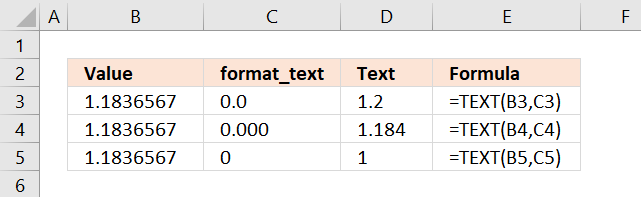You can round to thousands and millions and so on using the comma character.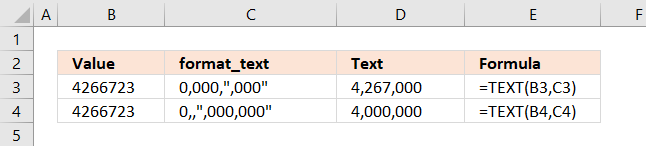### 3.2 Number formatting - thousands and millions

The comma symbol scales the number by a thousand, two comma symbols scale the number by a million (1000 * 1000 = 1,000,000).

K = 1,000 and M = 1,000,000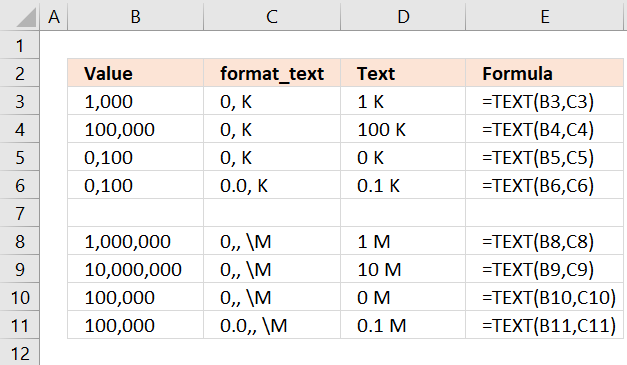The \ (backslash) symbol escapes the M character so Excel displays the letter M instead of converting the number into minutes.

The 0 (zero) symbol allows you to show leading zeros.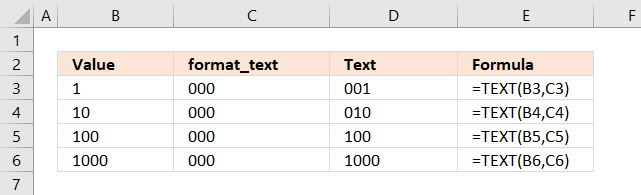### 3.4 Format positive, negative and zero values differently

The semicolon character lets you specify formatting code for positive, negative, zero values and text values. The picture below shows you these settings: 0.0;-0.00;"Zero";@

The first position is formatting code for positive values, here I have chosen 0.0. All positive values are rounded to one decimal.

The second position is affecting negative values, in this case, all negative values are rounded to two decimals.

The third position changes 0 to Zero and the fourth position shows the text value.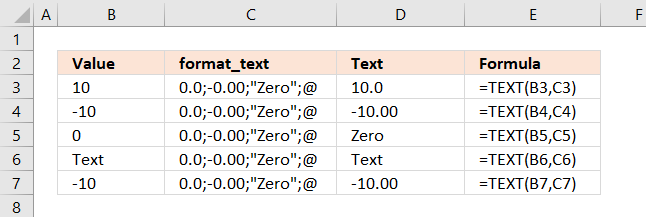## 4. Hide values using the TEXT function

### 4.1 How to hide positive numbers

Positive values are hidden if you leave out the first position.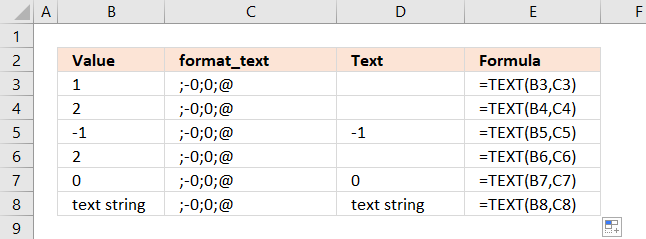### 4.2 How to hide negative numbers

Negative values are hidden if you leave the second position empty.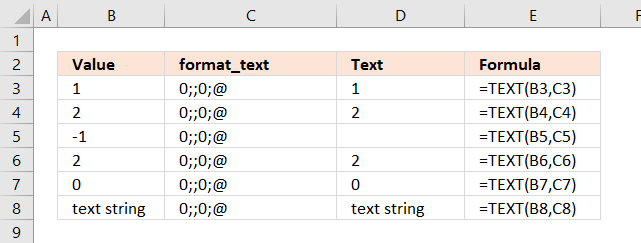### 4.3 How to hide zeros

If you leave the third position empty zeros are hidden.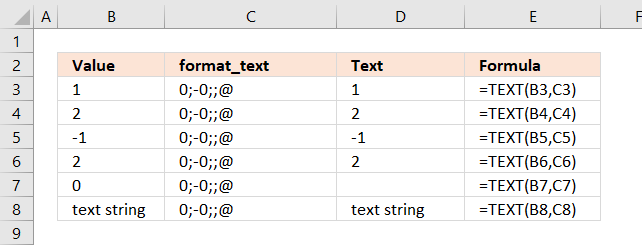### 4.4 How to hide text values

Omit the fourth position to hide text values.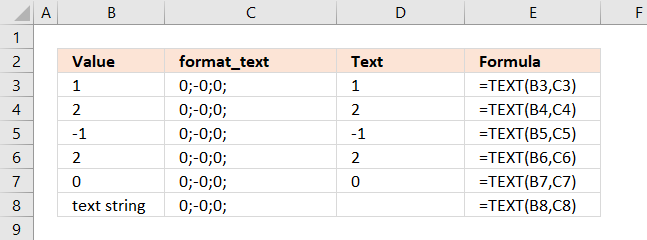## 5. Custom groups

Group 3 is assigned to values larger than 150, Group 2 is assigned to values larger than 100, Group 1 is assigned to all other values.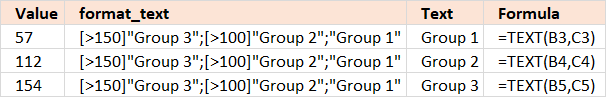## 6. Fractions

The division symbol lets you create fractions.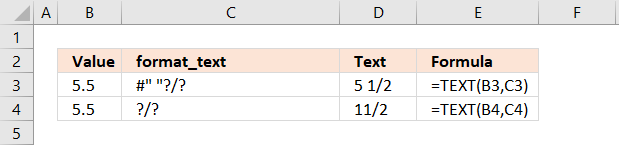## 7. Phone numbers

The 0 (zero) symbol lets you create phone numbers with leading 0's.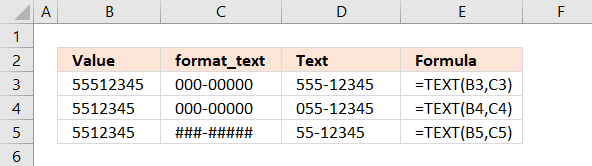## 8. Indents

Use the underscore character _ and then the character you want to use as an indent. The character is not shown.

The example below uses - (minus) as the indent character in the first position (positive numbers) to align negative and positive numbers. The first row is not aligned.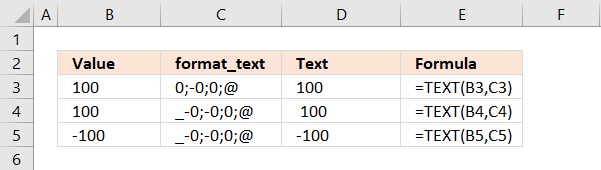## 9. Singular / Plural